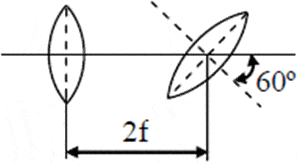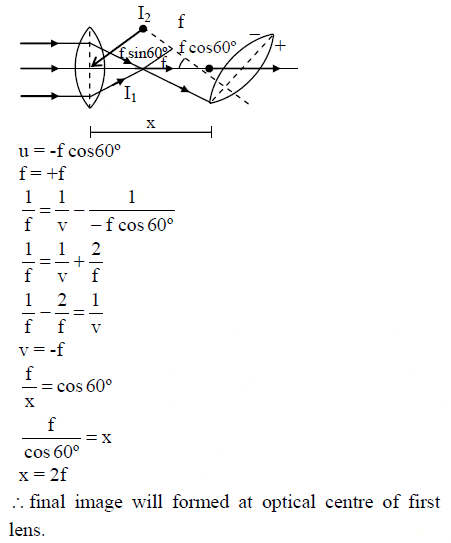Optics - Lens

Homework Statement

Two converging lenses of the same focal length f are separated by distance 2f. The axis of the second lens is inclined at angle θ = 60º with respect to the axis of the first lens. A parallel paraxial beam of light is incident from left side of the lens. Then:(A) Final image after all possible refraction will formed at optical centre of first lens
(B) Final image after all possible refraction will formed at optical centre of second lens
(C) Final image after all possible refraction will formed at distance f from second lens
(D) Final image after all possible refraction will formed at distance f from first lens

f = p.p'/p+p'

The Attempt at a Solution

Here the solution:But, I don't agree with this line:

f/x = cos 60, but I think the correct is x/f = cos 60, so x = f/2. But the answer is 2f. Anyone could see if i'm wrong ?

ehild
Homework Helper
You are right, the first image is at f/2 distance from the second lens.

ehild

Simon Bridge